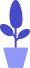# Python comparison operators

Estimated reading: 4 minutes 71 views

In this tutorial, let’s introduce a special integer type in Python – the Boolean type. Boolean type has only two values: True (true) or False (false). We call True and False Boolean values. A simple Boolean expression is shown below.

``````>>> thisIsBool=True
>>> print(thisIsBool)
True``````

In this example, we create a variable named thisIsBool and assign the boolean value True to it. On the next line, we print the thisIsBool variable, and the result is True.

Hint: In all high-level languages, there is a type of variable called Boolean, named after George Boolean.

George Boole was one of the most important British mathematicians in the 19th century. Because of his special contribution in symbolic logical operations, many computer languages ​​call logical operations Boolean operations, and their results are called Boolean values.

## Python comparison operators

Comparison operators compare two values ​​and get a boolean value. Comparison operators include: ==, !=, >, <, >=, and <=. These operators return True or False as the result of the comparison, depending on the value provided for them. Let’s look at these operators in detail.

#### equals (==)

If `==`the values ​​on both sides of the operator are the same, the result is True; if not, the result is False. For example, the expression 42==42 evaluates to True, but the expression 42==24 evaluates to False.

``````>>> 42==42
True
>>> 42==24
False``````

Tip: To determine if two values ​​are equal, remember to use two equals signs `==`, not one `=``==`means “are the values ​​on both sides equal?”, while `=`means “save the value on the right to the variable on the left”.

When `=`using , variable names must be placed on the left and values ​​must be placed on the right. And `==`is only used to compare two values ​​for equality, so it doesn’t matter which side the value is placed on.In the expression 42==42.0, although the two sides are of different types, one is an integer and the other is a floating-point number, but their values ​​are the same, so the result is still True.

``````>>> 42==42.00
True``````

But the expression 42==”42″ evaluates to False, because Python considers integers to be different from strings.

``````>>> 42=="42"
False``````

The return value of the expression “Johnson”==”johnson” is False, because the first letter of the two strings is different in case, so Python thinks they are two different strings.

``````>>> "Johnson"=="johnson"
False``````

The return value of the expression “Johnson”==”Johnson” is True because the two strings are identical.

``````>>> "Johnson"=="Johnson"
True``````

#### not equal (!=)

The opposite of the equal operator `==`is the not equal operator `!=`, which is composed of an exclamation mark and an equal sign, where the exclamation mark means not (or no, no, inverse, etc. means negation), which has similar meanings in many programming languages meaning.

We can use the unequal sign `!=`to determine whether the first value is not equal to the second value. For example, the expression 42!=”42″ is True, and “Johnson”!=”johnson” is also True.

``````>>> 42!="42"
True
>>> "Johnson"!="johnson"
True``````

#### Greater than (>) and greater than or equal to (>=)

You can use the greater than sign `>`to determine whether the first value is greater than the second value. For example, the expression 42>24 is true and the result is True; the expression 42>62 is not true and the result is False.

``````>>> 42>24
True
>>> 42>62
False``````

Use the greater than or equal sign `>=`to determine whether the first value is greater than or equal to the second value. If the first value is greater than the second value, the result of the greater than or equal sign is True; if the first value is equal to the second value, the result of the greater than or equal sign is also True.

``````>>> 42>=42
True``````

#### Less than (<) and less than or equal to (<=)

The opposite of the greater than operator `>`is the less than operator `<`, and the opposite of the greater than or equal operator `>=`is the less than or equal operator `<=`. Use the less than sign `<`to determine whether the first value is less than the second value; use the less than or equal sign `<=`to determine whether the first value is less than or equal to the second value. For details, please refer to the following examples, please pay attention to the comparison results:

``````>>> 42<62
True
>>> 42<=42
True
>>> 42<24
False``````